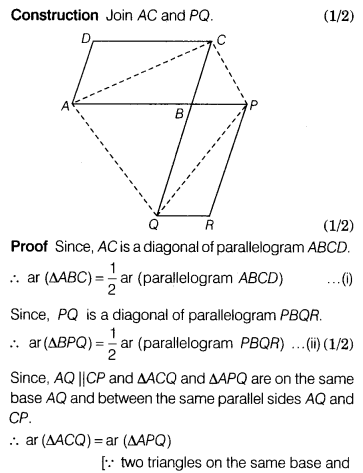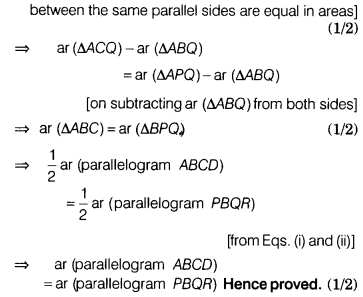# The side AB of a parallelogram ABCD is produced to any point P

The side AB of a parallelogram ABCD is produced to any point P. A line through A and parallel to CP meets CB produced at Q and then parallelogram PBQR is completed. Show that ar (parallelogram ABCD)= ar (parallelogram PBQR)

Given Side AB of a parallelogram ABCD is produced to any point P. A line through A and parallel to CP meets CB produced at Q and PBQR is a parallelogram.
To prove ar (parallelogram ABCD)= ar (parallelogram PBQR)